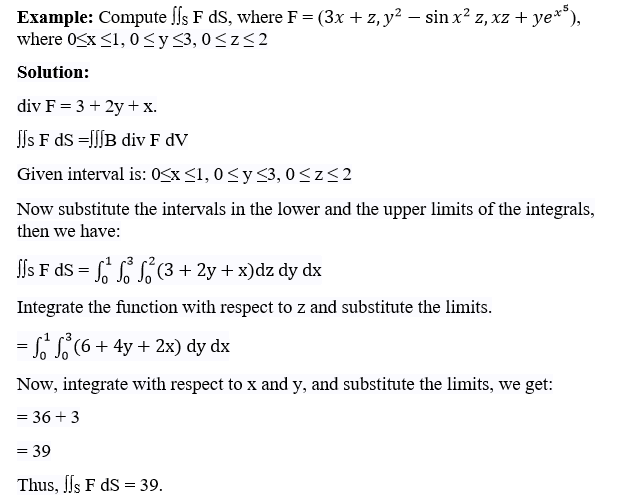# Divergence Theorem

In Calculus, the most important theorem is the “Divergence Theorem”. This theorem is used to solve many tough integral problems. It compares the surface integral with the volume integral. It means that it gives the relation between the two. In this article, you will learn the divergence theorem statement, proof, Gauss divergence theorem, and examples in detail.

## Divergence Theorem Statement

The divergence theorem states that the surface integral of the normal component of a vector point function “F” over a closed surface “S” is equal to the volume integral of the divergence of $$\vec{F}$$ taken over the volume “V” enclosed by the surface S. Thus, the divergence theorem is symbolically denoted as:

$$\iint_{v}\int \bigtriangledown \vec{F}. dV = \iint_{s}\vec{F}.\vec{n}.dS$$

## Divergence Theorem Proof

The divergence theorem-proof is given as follows:

Assume that “S” be a closed surface and any line drawn parallel to coordinate axes cut S in almost two points. Let S1 and S2 be the surface at the top and bottom of S. These are represented by z=f(x,y)and z=ϕ(x,y) respectively.

$$\vec{F}=F_{1}\vec{i}+F_{2}\vec{j}+F_{3}\vec{k}$$, then we have

$$\int \int \int \frac{\partial F_{3}}{\partial z}dV =\int \int \int \frac{\partial F_{3}}{\partial z} dx dy dz$$

$$\iint_{R}\left [ \int_{z =\Phi (x,y)}^{z =f(x,y)} \frac{\partial F_{3}}{\partial z} \right ]dx dy$$

$$\iint_{R} [F_{3}(x,y,z)]z = f(x, y)z =\Phi (x,y)dx dy$$

$$\iint_{R} [F_{3}(x,y,f)- F_{3}(x, y,\Phi )]dx dy$$ ——(1)

So, for the upper surface S2,

$$\frac{dy}{dx}= cos\gamma _{2}dS = \vec{k}.\vec{n_{2}}dS$$

Since the normal vector n2 to S2 makes an acute angle $$\gamma _{2}$$ with $$\vec{k}$$ vector,

$$dx dy = -cos \gamma _{2}dS_{1} = -\vec{k}. \vec{n}. dS_{1}$$

Since the normal vector n1 to S1 makes an obtuse angle $$\gamma _{1}$$ with $$\vec{k}$$ vector, then

$$\iint_{R}F_{3}(x,y,z)dxdy =\iint_{s_{2}}F_{3}\vec{k}.\vec{n_{2}}dS_{2}$$ —-(2)

$$\iint_{R}F_{3}(x,y,\Phi )dxdy =\iint_{s_{1}}F_{3}\vec{k}.\vec{n_{1}}dS_{1}$$ —-(3)

Now, the expression (1) can be written as:

$$\iint_{R}F_{3}(x,y,z)dxdy – \iint_{R}F_{3}(x,y,\Phi )dxdy$$ —-(4)

Now, substitute (2) and (3) in (4)

$$\iint_{s_{2}}F_{3}\vec{k}.\vec{n_{2}}dS_{2} – \iint_{s_{1}}F_{3}\vec{k}.\vec{n_{1}}dS_{1}$$

Thus, the above expression can be written as,

$$\iint_{s}F_{3}\vec{k}.\vec{n}dS$$

Similarly, projecting the surface S on the coordinate plane, we get

$$\int \int \int \frac{\partial F_{3}}{\partial z}dV = \int \int F_{3}\vec{k}.\vec{n}dS$$

$$\int \int \int \frac{\partial F_{2}}{\partial y}dV = \int \int F_{2}\vec{j}.\vec{n}dS$$

$$\int \int \int \frac{\partial F_{1}}{\partial x}dV = \int \int F_{1}\vec{i}.\vec{n}dS$$

Now, add the above all three equations, we get:

$$\iint_{v}\int \left [ \frac{\partial F_{1}}{\partial x} + \frac{\partial F_{2}}{\partial y}+ \frac{\partial F_{3}}{\partial z}\right ]dV =\iint_{s}[F_{1}\vec{i} +F_{2}\vec{j}+F_{3}\vec{k}]. \vec{n}.dS$$

Thus, the divergence theorem can be written as:

$$\iint_{v}\int \bigtriangledown \vec{F}. dV = \iint_{s}\vec{F}.\vec{n}.dS$$

Hence, proved.

### Divergence Theorem Example

Given below is an example for the divergence theorem.For more theorems and concepts in Maths concepts, visit BYJU’S – The Learning App and download the app to get the videos to learn with ease.